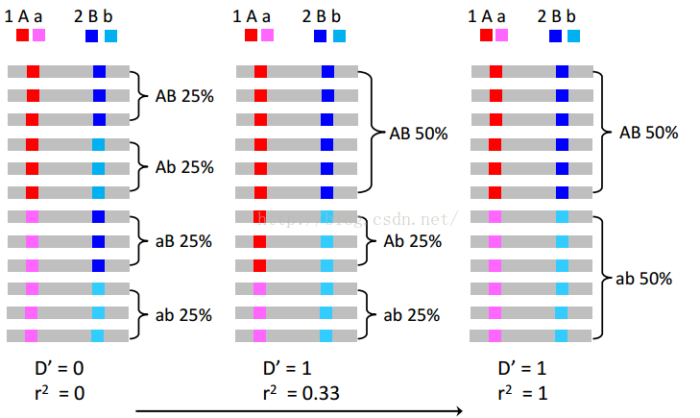# 连锁不平衡的计算方法

D是LD的基本单位，度量观察到的单倍型频率与平衡状态下期望频率的偏差，算法如下：

D=P(AB)-P(A)*P(B);

P(AB)表示实际观察到的AB频率，P(A)*P(B)表示AB频率的期望值。（如果发生连锁不平衡，实际观测到的AB频率肯定不等于AB频率的期望值）

D'=D/Dmax

r2=D*D/(P(A)P(a)P(B)P(b))

1 r2的期望值和有效种群大小和重组系数相关，r2=1/(1+4NeC),其中Ne是有小种群大小，C是重组系数。

2 r2有很好的取样特性，样本量和r2的乘积就是所观察到的关联水平概率对应的卡方值。在检测snp和致病位点之间的关联时，如果要达到同样的统计效力，所需要的样本量要增大1/r2倍。例如，假设snp1与疾病相关，我们要对它附近的snp2进行基因分型，他们之间的LD系数r2=0.5，为了达到与snp1位点检测相同的统计效力，必须把样本增加2倍。

3 与D'相比，在同样长度的染色体范围内，r2往往更低，这个特性能够帮助我们找到更精度的基因定位。1 P(A)=0.5, P(a)=0.5, P(B)=0.5, P(b)=0.5

D=0.25-0.5*0.5=0

D'=r2=0

2  P(A)=0.75, P(a)=0.25, P(B)=0.5, P(b)=0.5

D=0.5-0.75*0.25=0.125

D'=0.125/min{0.75*0.5, 0.25*0.5}=1

r2=0.125*0.125/(0.75*0.25*0.5*0.5)=0.33

3 P(A)=0.5, P(a)=0.5, P(B)=0.5, P(b)=0.5

D=0.5-0.5*0.5=0.25

D'=0.25/min{0.5*0.5, 0.5*0.5}=1

r2=0.25*0.25/(0.5*0.5*0.5*0.5)=1

• 版权声明 本文源自 -麦_子- 整理 发表
• 转载请务必保留本文链接：https://www.plob.org/article/21675.html